Most Affordable JEE | NEET | 8,9,10 Preparation by Kota's Top IITian Doctor Faculties

# NCERT Solutions for Class 11 Physics chapter 10 - Mechanical Properties of Fluids - Free PDF download`
Hey, are you a class 11 student and looking for ways to download NCERT Solutions for Class 11 Physics chapter 10 "Mechanical Properties of Fluids"? If yes. Then read this post till the end.

In this article, we have listed NCERT solution for class 11 Physics Chapter 10 in PDF that are prepared by Kota’s top IITian’s Faculties by keeping Simplicity in mind.

If you want to learn and understand class 11 Physics chapter 10 "Mechanical Properties of Fluids" in an easy way then you can use these solutions PDF.

NCERT Solutions helps students to Practice important concepts of subjects easily. Class 11 Physics solutions provide detailed explanations of all the NCERT questions that students can use to clear their doubts instantly.

If you want to score high in your class 11 Physics Exam then it is very important for you to have a good knowledge of all the important topics, so to learn and practice those topics you can use eSaral NCERT Solutions.

So, without wasting more time Let’s start.

### Download The PDF of NCERT Solutions for Class 11 Physics Chapter 10 "Mechanical Properties of Fluids"

Question 1 Explain why
(a) The blood pressure in humans is greater at the feet than at the brain
(b) Atmospheric pressure at the height of about $6 \mathrm{~km}$ decreases to nearly half of its value at the sea level, though the height of the atmosphere is more than $100 \mathrm{~km}$
(c) Hydrostatic pressure is a scalar quantity even though the pressure is force divided by area.

Solution: The pressure of a liquid is given by the relation:
$\mathrm{P}=\mathrm{h} \rho \mathrm{g}$ Where
$\mathrm{P}=$ Pressure
$\mathrm{h}=$ Height of the liquid column
$\rho=$ Density of the liquid
$\mathrm{g}=$ Acceleration due to the gravity (a) From the above expression of pressure, we can say that pressure is directly proportional to the height. Hence, the blood pressure in human depends on the height of the blood column in the body and blood column is more at the feet than at the brain. Hence, we can say that the blood pressure at the feet is more than at the brain.
(b) The density of air is the maximum near the sea level. The density of air decreases with an increase in height from the surface. At the height of about $6 \mathrm{~km}$, density decreases to nearly half of its value at the sea level. Atmospheric pressure is proportional to density. Hence, at the height of $6 \mathrm{~km}$ from the surface, it decreases to nearly half of its value at the sea level.
(c) When force is applied on a liquid, the effect of pressure in the liquid is transmitted in all directions. Hence, hydrostatic pressure does not have a fixed direction, and it is a scalar physical quantity.

$Question 2$ Explain why
(a) The angle of contact of mercury with glass is obtuse, while that of water with glass is acute.
(b) Water on a clean glass surface tends to spread out while mercury on the same surface tends to form drops. (Put differently, water wets glass while mercury does not.)
(c) The surface tension of a liquid is independent of the area of the surface
(d) Water with detergent dissolved in it should have small angles of contact.
(e) A drop of liquid under no external forces is always spherical in shape

Solution: (a) The Angle of contact $(\theta)$ is the angle between the tangent to the liquid surface at the point of contact and the surface inside the liquid. The angle of contact $(\theta)$ is as shown in the given figure.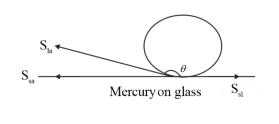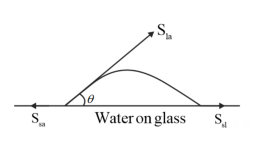$\mathrm{S}_{\mathrm{la}}, \mathrm{S}_{\mathrm{sa}}$, and $\mathrm{S}_{\mathrm{sl}}$ are the interface tensions between the solid-air, liquidair and solid-liquid interfaces. At the line of contact, the surface forces between the three media must be in equilibrium, i.e.,
$\cos \theta=\frac{\mathrm{S}_{\mathrm{sa}}-\mathrm{S}_{\mathrm{sl}}}{\mathrm{S}_{\mathrm{la}}}$
The angle of contact $\theta$ is obtuse if $\mathrm{S}_{\mathrm{sa}}<\mathrm{S}_{\mathrm{la}}$ (from the figure of mercury on glass).
This angle is acute if $\mathrm{S}_{\mathrm{sl}}<\mathrm{S}_{\mathrm{la}}$ (from the figure of water on glass).
(b) Mercury molecules, which make an obtuse angle with glass, have a strong force of attraction between themselves and a weak force of attraction toward solids. Hence, they tend to form drop while water molecules make acute angles with glass. They have a weak force of attraction between themselves and a strong force of attraction toward solids. Hence, they have the possibility to spread out.
(c) Surface tension is the force acting per unit length at the interface between the plane of a liquid and any other surface. This force does not depend on the area of the liquid surface. Hence, surface tension is also independent of the area of the liquid surface.
(d) Water with detergent dissolved in it has small angles of contact $(\theta)$ because, for a small $\theta$, there is a fast capillary rise of the detergent in the cloth. The capillary rise of a liquid is directly proportional to the cosine of the angle of contact $(\theta)$. If $\theta$ is small, then $\cos \theta$ will be large, and the rise of the detergent water in the cloth will be fast.
(e) A liquid always wants to acquire the minimum surface area because of the presence of surface tension. For a given volume, the surface area of a sphere is the minimum. Hence if there is no external force on the liquid drop, it will always take spherical shape.

$Question3$ Fill in the blanks using the word(s) from the list appended with each statement:
(a) Surface tension of liquids generally with temperatures (increases /decreases)
(b) Viscosity of gases with temperature, whereas viscosity of liquids with temperature (increases / decreases)
(c) For solids with an elastic modulus of rigidity, the shearing force is proportional to while for fluids it is proportional to (shear strain / rate of shear strain)
(d) For a fluid in a steady flow, the increase in flow speed at a constriction follows from while the decrease of pressure follows from (conservation of mass / Bernoulli's principle)
(e) For the model of a plane in a wind tunnel, turbulence occurs at a speed for turbulence for an actual plane (greater / smaller)

Solution: (a) Decreases
The surface tension of a liquid is inversely proportional to temperature. Surface tension is a property that arises due to the intermolecular forces of attraction among the molecules of the liquid. The decrease in surface tension with an increase of temperature is due to the fact that with an increase in temperature, the kinetic energy of the molecules increases. Therefore, the intermolecular attraction decreases and surface tension will also decrease.
(b) Increases; decreases
Most fluids offer resistance to their motion. This is like internal mechanical friction, known as viscosity. The viscosity of gases increases with temperature, while the viscosity of liquids decreases with temperature.
(c) Shear strain; Rate of shear strain
According to the elastic modulus of rigidity for solids, the shearing force is proportional to the shear strain. According to the elastic modulus of rigidity for fluids, the shearing force is proportional to the rate of shear strain.
(d) Conservation of mass, Bernoulli's principle
For a steady-flowing fluid, an increase in its flow speed at a constriction follows the continuity equation while the decrease of pressure is due to Bernoulli's principle.
(e) Greater
For the model of a plane in a wind tunnel, turbulence occurs at a greater speed than for an actual plane because it follows Bernoulli's principle, and different Reynolds' numbers are associated with the motions of the two planes.

$Question4$ Explain why
(a) To keep a piece of paper horizontal, you should blow over, not under, it
(b) When we try to close a water tap with our fingers, fast jets of water gush through the openings between our fingers
(c) The size of the needle of a syringe controls flow rate better than the thumb pressure exerted by a doctor while administering an injection
(d) A fluid flowing out of a small hole in a vessel results in a backward thrust on the vessel
(e) A spinning cricket ball in air does not follow a parabolic trajectory

Solution: (a) When we blow over a piece of paper, the velocity of air above the paper becomes more than that below it. Since, the kinetic energy of air above the paper increases, so in accordance with Bernoulli's theorem $\left(P+\frac{\rho v^{2}}{2}\right.$ = constant), its pressure decreases. Because of this paper will experience an upthrust, and this will balance the weight of paper which will keep it straight.
(b) According to the equation of continuity: Area $\times$ Velocity $=$ Constant
For a smaller opening, the velocity of flow of a fluid is greater than it is when the opening is bigger. When we try to close a tap of water with our fingers, fast jets of water gush through the openings between our fingers. This is because very small openings are left for the water to flow out of the pipe.
(c) The small opening of a syringe needle controls the velocity of the blood flowing out. It follows the equation of continuity. At the constriction point of the syringe system, the flow rate suddenly increases to a high value for a constant thumb pressure applied.
(d) When a fluid flows out from a small hole in a vessel, the vessel receives a backward thrust. A fluid flowing out from a small hole has a large velocity according to the equation of continuity: Area $\times$ Velocity = Constant
According to the law of conservation of momentum, the vessel gains a backward velocity because there are no external forces acting on the system.
(e) If a ball is moving from left to right and also spinning about a horizontal axis perpendicular to the direction of motion as shown in fig. then relative to the ball, air will be moving from right to left.
The resultant velocity of air above the ball will be $(v+r \omega)$ while below it $(v-r \omega)$. So. in accordance with Bernoulli's principal pressure above the ball will be less than below it. Due to this difference of pressure, an upward force will act on the ball, and hence the ball will deviate from its usual path $O A_{0}$ and will hit the ground at $A_{1}$ following the path $O A_{1}$,
i.e., if a ball is thrown with back-spin, the pitch will curve less sharply prolonging the flight.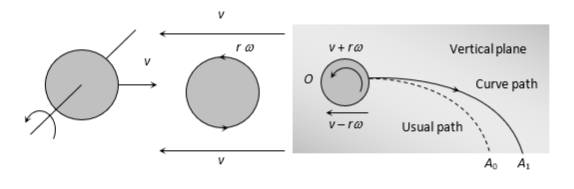Similarly, if the spin is clockwise, i.e., the ball is thrown with top-spin, the force due to pressure difference will act in the direction of gravity, and so the pitch will curve more sharply shortening the flight. Furthermore, if the ball is spinning about a vertical axis, the curving will be sideways as shown in producing the so-called out swing or in swing, and it will not follow a parabolic path.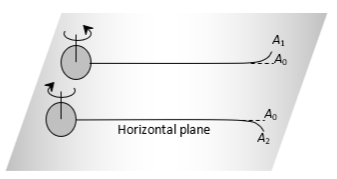Question.5 A $50 \mathrm{~kg}$ girl wearing high heel shoes balances on a single heel. The heel is circular with a diameter of $1.0 \mathrm{~cm}$. What is the pressure exerted by the heel on the horizontal floor?

Solution: Given that,
Mass of the girl, $\mathrm{m}=50 \mathrm{~kg}$
The diameter of the heel, $\mathrm{d}=1 \mathrm{~cm}=0.01 \mathrm{~m}$
The radius of the heel, $\mathrm{r}=\frac{\mathrm{d}}{2}=0.005 \mathrm{~m}$
Area of the heel $=\pi r^{2}=\pi(0.005)^{2}=7.85 \times 10^{-5} \mathrm{~m}^{2}$
Force exerted by the heel on the floor equal to the weight of the heel:
$\mathrm{F}=\mathrm{mg}=50 \times 9.8=490 \mathrm{~N}$
The pressure exerted by the heel on the floor:
$\mathrm{P}=\frac{\text { Force }}{\text { Area }}=\frac{490}{7.85 \times 10^{5}}$
$=6.24 \times 10^{6} \mathrm{~N} \mathrm{~m}^{-2}$
Therefore, the pressure exerted by the heel on the horizontal floor is $6.24 \times 10^{6} \mathrm{~N} \mathrm{~m}^{-2}$

$Question.6$ Torricelli's barometer used mercury. Pascal duplicated it using French wine of density $984 \mathrm{~kg} \mathrm{~m}^{-3}$. Determine the height of the wine column for normal atmospheric pressure.

Solution. Solution:
We know that density of mercury, $\rho_{1}=13.6 \times 10^{3} \mathrm{~kg} / \mathrm{m}^{3}$
Height of the mercury column, $\mathrm{h}_{1}=0.76 \mathrm{~m}$
The density of French wine, $\rho_{2}=984 \mathrm{~kg} / \mathrm{m}^{3}$
Height of the French wine column $=\mathrm{h}_{2}$
Acceleration due to gravity, $\mathrm{g}=9.8 \mathrm{~m} / \mathrm{s}^{2}$
The pressure in both columns is equal, i.e.,
Pressure in the mercury column $=$ Pressure in the French wine column
$\rho_{1} \mathrm{~h}_{1} \mathrm{~g}=\rho_{2} \mathrm{~h}_{2} \mathrm{~g}$
$\mathrm{h}_{2}=\frac{\rho_{1} \mathrm{~h}_{1}}{\rho_{2}}$
$\mathrm{h}_{2}=\frac{13.6 \times 10^{3} \times 0.76}{984} \mathrm{~m}$
$h_{2}=10.5 \mathrm{~m}$
Hence, the height of the French wine column for normal atmospheric pressure is $10.5 \mathrm{~m}$.

Question.7 A vertical off-shore structure is built to withstand maximum stress of $10^{9} \mathrm{~Pa}$. Is the structure suitable for putting up on top of an oil well in the ocean ? Take the depth of the ocean to be roughly $3 \mathrm{~km}$, and ignore ocean currents.

Solution. Given that the maximum allowable stress for the structure, $\mathrm{P}=10^{9} \mathrm{~Pa}$
Depth of the ocean, $\mathrm{d}=3 \mathrm{~km}=3 \times 10^{3} \mathrm{~m}$
The density of water, $\rho=10^{3} \mathrm{~kg} / \mathrm{m}^{3}$
Acceleration due to gravity, $\mathrm{g}=9.8 \mathrm{~m} / \mathrm{s}^{2}$
The pressure exerted because of the sea water at depth, $\mathrm{d}=\rho \times \mathrm{d} \times \mathrm{g}$ $\mathrm{P}=3 \times 10^{3} \times 10^{3} \times 9.8=2.94 \times 10^{7} \mathrm{~Pa}$
The maximum allowable stress for the structure $\left(10^{9} \mathrm{~Pa}\right)$ is greater than the pressure of the sea water $\left(2.94 \times 10^{7} \mathrm{~Pa}\right)$. The pressure exerted by the ocean is less than the pressure that the structure can withstand. Hence, the structure is suitable for putting up on top of an oil well in the ocean.

$Question.8$ A hydraulic automobile lift is designed to lift cars with a maximum mass of $3000 \mathrm{~kg}$. The area of cross-section of the piston carrying the load is $425 \mathrm{~cm}^{2}$. What maximum pressure would the smaller piston have to bear?

Solution. Given that the maximum mass of a car that can be lifted, $\mathrm{m}=3000 \mathrm{~kg}$
Area of a cross-section of the load-carrying piston, $A=425 \mathrm{~cm}^{2}=$ $425 \times 10^{-4} \mathrm{~m}^{2}$
The maximum force exerted by the load,
$\mathrm{F}=\mathrm{mg}=3000 \times 9.8=29400 \mathrm{~N}$
The maximum pressure exerted on the load-carrying piston, $\mathrm{P}=\frac{\text { Force }}{\text { Area }}$
$\mathrm{P}=\frac{29400}{425 \times 10^{-4}} \mathrm{~Pa}=6.917 \times 10^{5} \mathrm{~Pa}$
The pressure is transmitted equally in all directions in a liquid. Therefore, the maximum pressure that the smaller piston would have to bear is $6.917 \times$ $10^{5} \mathrm{~Pa}$

$Question.9$ A U-tube contains water and methylated spirit separated by mercury. The mercury columns in the two arms are in level with $10.0 \mathrm{~cm}$ of water in one arm and $12.5 \mathrm{~cm}$ of spirit in the other. What is the specific gravity of spirit?

Solution. We know that specific gravity is defined as the ratio of the density of a substance to the density of water.
The given system of water, mercury, and methylated spirit are shown as follows: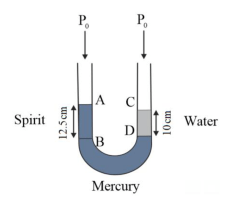Height of the spirit column, $h_{1}=12.5 \mathrm{~cm}=0.125 \mathrm{~m}$
Height of the water column, $\mathrm{h}_{2}=10 \mathrm{~cm}=0.1 \mathrm{~m}$
$\mathrm{P}_{0}=$ Atmospheric pressure
$\rho_{1}=$ Density of spirit
$\rho_{2}=$ Density of water
The pressure at point $\mathrm{B}=\mathrm{P}_{0}+\mathrm{h}_{1} \rho_{1} \mathrm{~g}$
The pressure at point $\mathrm{D}=\mathrm{P}_{0}+\mathrm{h}_{2} \rho_{2} \mathrm{~g}$
The pressure at points $\mathrm{B}$ and $\mathrm{D}$ is the same.
$\mathrm{P}_{0}+\mathrm{h}_{1} \rho_{1} \mathrm{~g}=\mathrm{P}_{0}+\mathrm{h}_{2} \rho_{2} \mathrm{~g}$
$\frac{\rho_{1}}{\rho_{2}}=\frac{\mathrm{h}_{2}}{\mathrm{~h}_{1}}$
$=\frac{10}{12.5}=0.8$
Therefore, the specific gravity of spirit is $0.8$.

$Question.10$ In the previous problem, if $15.0 \mathrm{~cm}$ of water and spirit each are further poured into the respective arms of the tube, what is the difference in the levels of mercury in the two arms ? (Specific gravity of mercury $=13.6$ )

Solution. Height of the water column, $\mathrm{h}_{1}=10+15=25 \mathrm{~cm}$
Height of the spirit column, $\mathrm{h}_{2}=12.5+15=27.5 \mathrm{~cm}$
The density of water, $\rho_{1}=1 \mathrm{~g} \mathrm{~cm}^{-3}$
The density of spirit, $\rho^{2}=0.8 \mathrm{~g} \mathrm{~cm}^{-3}$
The density of mercury $=13.6 \mathrm{~g} \mathrm{~cm}^{-3}$
Let $\mathrm{h}$ be the difference between the levels of mercury in the two arms.
The pressure exerted by height h, of the mercury column:
$\mathrm{P}^{\prime}=\mathrm{h} \rho \mathrm{g}=\mathrm{h} \times 13.6 \times \mathrm{g} \ldots \ldots \ldots \ldots . .$ (i)
Difference between the pressures exerted by water and spirit:
$=h_{1} \rho_{1} g-h_{1} \rho_{1} g$
$=g(25 \times 1-27.5 \times 0.8)=3 g \ldots \ldots \ldots$ (ii)
Equating equations (i) and (ii), we get:
$13.6 h g=3 g$
$h=0.220588 \approx 0.221 \mathrm{~cm}$
Hence, the difference between the levels of mercury in the two arms is $0.221 \mathrm{~cm}$

Question.11 Can Bernoulli's equation be used to describe the flow of water through a rapid in a river? Explain.

Solution:No
Bernoulli’s equation cannot be used to describe the flow of water through arapid in a river because of the turbulent flow of water. This principle can only be applied to a streamline flow.

Qolution.12 Does it matter if one uses gauge instead of absolute pressures in applying Bernoulli's equation? Explain.

Solution:No
It does not matter if one uses gauge pressure instead of absolute pressure while applying Bernoulli's equation. For applying Bernoulli's equation between two points, pressure should be different at two points.

Question.13 Glycerine flows steadily through a horizontal tube of the length of $1.5 \mathrm{~m}$ and radius $1.0 \mathrm{~cm} .$ If the amount of glycerine collected per second at one end is $4.0 \times 10^{-3} \mathrm{~kg} \mathrm{~s}^{-1}$, what is the pressure difference between the two ends of the tube ? (Density of glycerine $=1.3 \times 10^{3} \mathrm{~kg} \mathrm{~m}^{-3}$ and viscosity of glycerine $=$ $0.83 \mathrm{~Pa} \mathrm{~s}$ ). [You may also like to check if the assumption of laminar flow in the tube is correct]

Solution: Given that,
Length of the horizontal tube, $l=1.5 \mathrm{~m}$
Radius of the tube, $\mathrm{r}=1 \mathrm{~cm}=0.01 \mathrm{~m}$
The diameter of the tube, $\mathrm{d}=2 \mathrm{r}=0.02 \mathrm{~m}$
Glycerine is flowing at a rate of $4.0 \times 10^{-3} \mathrm{~kg} \mathrm{~s}^{-1}$.
$\mathrm{M}=4.0 \times 10^{-3} \mathrm{~kg} \mathrm{~s}^{-1}$
The density of glycerine, $\rho=1.3 \times 10^{3} \mathrm{~kg} \mathrm{~m}^{-3}$
The viscosity of glycerine, $\eta=0.83$ Pa s The volume of glycerine flowing per sec, $V=\frac{M}{\rho}$
$V=\frac{4.0 \times 10^{-3}}{1.3 \times 10^{3}} \mathrm{~m}^{3} \mathrm{~s}^{-1}$
$\Rightarrow V=3.08 \times 10^{-6} \mathrm{~m}^{3} \mathrm{~s}^{-1}$
From the Poiseville's formula, we have the relation for the rate of flow:
$\mathrm{V}=\frac{\pi \mathrm{Pr}^{4}}{8 \eta \mathrm{l}}$
Where $\mathrm{P}$ is the pressure difference between the two ends of the tube.
$\therefore \mathrm{P}=\frac{\mathrm{V} 8 \eta \mathrm{l}}{\pi \mathrm{r}^{4}}$
$\mathrm{P}=\frac{3.08 \times 10^{-6} \times 8 \times 0.83 \times 1.5}{\pi \times(0.01)^{4}} \mathrm{~Pa}$
$\mathrm{P}=9.8 \times 10^{2} \mathrm{~Pa}$
Reynolds number is given by the relation:
$\mathrm{R}=\frac{4 \rho \mathrm{V}}{\pi \mathrm{d} \eta}$
$=\frac{4 \times 1.3 \times 10^{3} \times 3.08 \times 10^{-6}}{\pi \times(0.02) \times 0.83}=0.3$
Reynolds' number is about $0.3$.
We know that if Reynolds number is less than 2000 , then the flow will be laminar.
Hence, the flow is laminar.

$Question.14$ In a test experiment on a model aeroplane in a wind tunnel, the flow speeds on the upper and lower surfaces of the wing are $70 \mathrm{~m} \mathrm{~s}^{-1}$ and $63 \mathrm{~m} \mathrm{~s}^{-1}$ respectively. What is the lift on the wing if its area is $2.5 \mathrm{~m}^{2}$ ? Take the density of air to be $1.3 \mathrm{~kg} \mathrm{~m}^{-3}$. Solution: Given that
Speed of wind on the upper surface of the wing, $V_{1}=70 \mathrm{~m} / \mathrm{s}$
Speed of wind on the lower surface of the wing, $V_{2}=63 \mathrm{~m} / \mathrm{s}$
Area of the wing, $A=2.5 \mathrm{~m}^{2}$
The density of air, $\rho=1.3 \mathrm{~kg} \mathrm{~m}^{-3}$
Apply Bernoulli's theorem between the upper and lower surface of the model aeroplane; We have the relation:
$P_{1}+\frac{1}{2} \rho V_{1}^{2}=P_{2}+\frac{1}{2} \rho V_{2}^{2}$
$P_{2}-P_{1}=\frac{1}{2} \rho\left(V_{1}^{2}-V_{2}^{2}\right)$
Where,
$P_{1}=$ Pressure on the upper surface of the wing
$P_{2}=$ Pressure on the lower surface of the wing
Lift to the aeroplane is equal to the pressure difference between the upper and lower surfaces.
Lift on the wing $=\left(\mathrm{P}_{2}-\mathrm{P}_{1}\right) \mathrm{A}$
$\Rightarrow$ Lift on the wing
$=\frac{1}{2} \rho\left(V_{1}^{2}-V_{2}^{2}\right)$
$\Rightarrow$ Lift on the wing
$=\frac{1}{2} 1.3 \times\left((70)^{2}-(63)^{2}\right) \times 2.5 \mathrm{~N}$
$\Rightarrow$ Lift on the wing $=1512.87 \mathrm{~N}$
$\Rightarrow$ Lift on the wing $=1.51 \times 10^{3} \mathrm{~N}$
Therefore, the lift on the wing of the aeroplane is $1.51 \times 10^{3} \mathrm{~N}$.

$Question.15$ Figures (a) and (b) refer to the steady flow of a (non-viscous) liquid. Which of the two figures is incorrect? Why?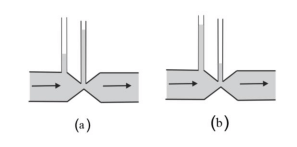Solution: (a) Take the case given in figure
(b)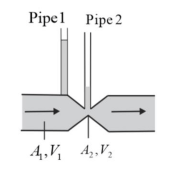Where,
$A_{1}=$ Area of pipe 1
$A_{2}=$ Area of pipe 2
$\mathrm{V}_{1}=$ Speed of the fluid in pipe 1
$\mathrm{V}_{2}=$ Speed of the fluid in pipe 2
From the law of continuity, we have:
$\mathrm{A}_{1} \mathrm{~V}_{1}=\mathrm{A}_{2} \mathrm{~V}_{2}$
When the area of the cross-section in the middle of the venturi meter is small, the speed of the flow of liquid through this cross-sectional area is more. According to Bernoulli's principle, if speed is more, then pressure is less.
The pressure is directly proportional to height. Hence, the level of water in pipe 2 is less. Therefore, the figure (a) is not possible.

$Question.16$ The cylindrical tube of a spray pump has a cross-section of $8.0 \mathrm{~cm}^{2}$ one end of which has 40 fine holes each of diameter $1.0 \mathrm{~mm}$. If the liquid flow inside the tube is $1.5 \mathrm{~m} \min ^{-1}$ what is the speed of ejection of the liquid through the holes ?

Solution:Given that,
Area of a cross-section of the spray pump, $A_{1}=8 \mathrm{~cm}^{2}=8 \times 10^{-4} \mathrm{~m}^{2}$
Number of holes, $\mathrm{n}=40$
The diameter of each hole, $\mathrm{d}=1 \mathrm{~mm}=1 \times 10^{-3} \mathrm{~m}$
Radius of each hole, $\mathrm{r}=\mathrm{d} / 2=0.5 \times 10^{-3} \mathrm{~m}$
Area of a cross-section of each hole,
$\mathrm{a}=\pi \mathrm{r}^{2}=\pi\left(0.5 \times 10^{-3}\right)^{2} \mathrm{~m}^{2}$
The total area of 40 holes, $A_{2}=\mathrm{n} \times \mathrm{a}$
$=40 \times \pi\left(0.5 \times 10^{-3}\right)^{2} \mathrm{~m}^{2}=31.41 \times$ $10^{-6} \mathrm{~m}^{2}$
Inside the tube, Speed of flow of liquid,
$V_{1}=1.5 \mathrm{~m} / \mathrm{min}=\frac{1.5}{60} \mathrm{~m} / \mathrm{s}=$
$0.025 \mathrm{~m} / \mathrm{s}$
Assume that speed of ejection of liquid through the holes $=V_{2}$
According to the law of continuity, we have:
$A_{1} V_{1}=A_{2} V_{2}$
$V_{2}=\frac{A_{1} V_{1}}{A_{2}}$
$V_{2}=\frac{8 \times 10^{-4} \times 0.025}{31.61 \times 10^{-6}}=0.633 \mathrm{~m} / \mathrm{s}$
Therefore, the speed of ejection of the liquid through the holes is $0.633 \mathrm{~m} / \mathrm{s}$.

$Question.17$ A U-shaped wire is dipped in a soap solution and removed. The thin soap film formed between the wire and the light slider supports a weight of $1.5 \times 10^{-2} \mathrm{~N}$ (which includes the small weight of the slider). The length of the slider is $30 \mathrm{~cm}$. What is the surface tension of the film?

Solution: Given that the soap film supports a weight of, $\mathrm{W}=1.5 \times 10^{-2} \mathrm{~N}$
Length of the slider, $1=30 \mathrm{~cm}=0.3 \mathrm{~m}$
We know that a soap film has two free surfaces.
$\therefore$ Total length $=2 \mathrm{l}=2 \times 0.3=0.6 \mathrm{~m}$
We know that surface tension, $\mathrm{S}=\frac{\text { Force }}{\text { length }}=\frac{\text { Weight }}{21}$
$\mathrm{S}=\frac{1.5 \times 10^{-2}}{0.6}=2.5 \times 10^{-2} \mathrm{~N} / \mathrm{m}$
Therefore, the surface tension of the film is $2.5 \times 10^{-2} \mathrm{~N} \mathrm{~m}^{-1}$.

$Question.18$ Figure (a) shows a thin liquid film supporting a small weight $=4.5 \times 10^{-2} \mathrm{~N}$. What is the weight supported by a film of the same liquid at the same temperature in Figure. (b) and (c)? Explain your answer physically.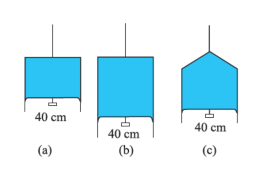Solution:
In case (a):
The length of the liquid film supported by the weight,
$\mathrm{l}=40 \mathrm{~cm}=0.4 \mathrm{~m}$
The weight supported by the film,
$\mathrm{W}=4.5 \times 10^{-2} \mathrm{~N}$
A liquid film has two free surfaces.
$\therefore$ Surface tension $=\frac{\mathrm{W}}{21}=\frac{4.5 \times 10^{-2}}{2 \times 0.4}$
$=5.625 \times 10^{-2} \mathrm{~N} \mathrm{~m}^{-1}$
In all the three figures, the liquid is the same and temperature is also the same.
Hence, the surface tension in figure (b) and figure (c) is the same as in figure (a), i.e.,
$5.625 \times 10^{-2} \mathrm{~N} \mathrm{~m}^{-1}$
Since the length of the film in all the cases is $40 \mathrm{~cm}$, the weight supported in each case is
$4.5 \times 10^{-2} \mathrm{~N}$

Question.19 What is the pressure inside the drop of mercury of radius $3.00 \mathrm{~mm}$ at room temperature ? The surface tension of mercury at that temperature $\left(20^{\circ} \mathrm{C}\right)$ is $4.65 \times 10^{-1} \mathrm{~N} \mathrm{~m}^{-1}$. The atmospheric pressure is $1.01 \times 10^{5} \mathrm{~Pa}$. Also give the excess pressure inside the drop.

Solution: Given that the radius of the mercury drop,
$\mathrm{r}=3.00 \mathrm{~mm}=3 \times 10^{-3} \mathrm{~m}$
The surface tension of mercury,
$\mathrm{S}=4.65 \times 10^{-1} \mathrm{~N} \mathrm{~m}^{-1}$

Atmospheric pressure,
$\mathrm{P}_{0}=1.01 \times 10^{5} \mathrm{~Pa}$
Assume total pressure inside the mercury drop is $\mathrm{P}$
$\therefore \mathrm{P}=$ Excess pressure inside mercury $+$ Atmospheric pressure
$\Rightarrow \mathrm{P}=\frac{2 \mathrm{~S}}{\mathrm{r}}+\mathrm{P}_{0}$
$\Rightarrow \mathrm{P}=\frac{2 \times 4.65 \times 10^{-1}}{3 \times 10^{-3}}+1.01 \times 10^{5} \mathrm{~Pa}$
$\Rightarrow \mathrm{P}=310 \mathrm{~Pa}$
Hence total pressure inside the mercury drop is $310 \mathrm{~Pa}$

$Question.20$ What is the excess pressure inside a bubble of soap solution of radius $5.00 \mathrm{~mm}$, given that the surface tension of soap solution at the temperature $\left(20^{\circ} \mathrm{C}\right)$ is $2.50 \times 10^{-2} \mathrm{~N} \mathrm{~m}^{-1} ?$ If an air bubble of the same dimension were formed at a depth of $40.0 \mathrm{~cm}$ inside a container containing the soap solution of relative density $1.20$, what would be the pressure inside the bubble ? (1 atmospheric pressure is $1.01 \times 10^{5} \mathrm{~Pa}$ ).

Solution: Given that the pressure inside the air bubble is $1.06 \times 10^{5} \mathrm{~Pa}$
The radius of a soap bubble,
$\mathrm{r}=5.00 \mathrm{~mm}=5 \times 10^{-3} \mathrm{~m}$
The surface tension of the soap solution,
$S=2.50 \times 10^{-2} \mathrm{~N} \mathrm{~m}^{-1}$
The relative density of the soap solution $=1.20$
The density of the soap solution,
$\rho=1.2 \times 10^{3} \mathrm{~kg} / \mathrm{m}^{3}$
Air bubble formed at depth,
$\mathrm{h}=40 \mathrm{~cm}=0.4 \mathrm{~m}$
The radius of the air bubble,
$\mathrm{r}=5 \mathrm{~mm}=5 \times 10^{-3} \mathrm{~m}$
1 atmospheric pressure $=1.01 \times 10^{5} \mathrm{~Pa}$
Acceleration due to gravity, $\mathrm{g}=9.8 \mathrm{~m} / \mathrm{s}^{2}$
Hence, the expression for the excess pressure inside the soap bubble is
$P=\frac{4 S}{r}$
$\Rightarrow P=\frac{4 \times 2.5 \times 10^{-2}}{5 \times 10^{-3}} \mathrm{~Pa}$
$\Rightarrow P=20 \mathrm{~Pa}$
Therefore, the excess pressure inside the soap bubble is $20 \mathrm{~Pa}$.
We know that excess pressure inside the air bubble is given by the relation:
$\mathrm{P}^{\prime}=\frac{2 \mathrm{~S}}{\mathrm{r}}$
$\mathrm{P}^{\prime}=\frac{2 \times 2.5 \times 10^{-2}}{5 \times 10^{-3}} \mathrm{~Pa}$
$\mathrm{P}^{\prime}=10 \mathrm{~Pa}$
Therefore, the excess pressure inside the air bubble is $10 \mathrm{~Pa}$.
The total pressure inside the air bubble at a depth of $0.4 \mathrm{~m}$ is
$=$ Atmospheric pressure $+\mathrm{h} \rho \mathrm{g}+\mathrm{P}^{\prime}$
$=1.01 \times 10^{5}+0.4 \times 1.2 \times 10^{3} \times 9.8+10$
$=1.057 \times 10^{5} \mathrm{~Pa}$
$=1.06 \times 10^{5} \mathrm{~Pa}$
$=1.01 \times 10^{5}+0.4 \times 1.2 \times 10^{3} \times 9.8+10$
$=1.057 \times 10^{5} \mathrm{~Pa}$
$=1.06 \times 10^{5} \mathrm{~Pa}$
Therefore, the pressure inside the air bubble is $1.06 \times 10^{5} \mathrm{~Pa}$

$Solution.21$ A tank with a square base of area $1.0 \mathrm{~m}^{2}$ is divided by a vertical partition in the middle. The bottom of the partition has a small-hinged door of area $20 \mathrm{~cm}^{2}$. The tank is filled with water in one compartment, and an acid (of relative density 1.7) in the other, both to a height of $4.0 \mathrm{~m}$. compute the force necessary to keep the door close.

Solution: The base area of the given tank, $A=1.0 \mathrm{~m}^{2}$
Area of the hinged door, $a=20 \mathrm{~cm}^{2}=20 \times 10^{-4} \mathrm{~m}^{2}$
The density of water, $\rho_{1}=10^{3} \mathrm{~kg} / \mathrm{m}^{3}$
The density of acid, $\rho_{2}=1.7 \times 10^{3} \mathrm{~kg} / \mathrm{m}^{3}$
Height of the water column, $h_{1}=4 \mathrm{~m}$
Height of the acid column, $h_{2}=4 \mathrm{~m}$
Acceleration due to gravity, $\mathrm{g}=9.8 \mathrm{~m} / \mathrm{s}^{2}$
Pressure due to water is given as:
$P_{1}=h_{1} \rho_{1} g$
$\Rightarrow P_{1}=4 \times 10^{3} \times 9.8 \mathrm{~Pa}$
$\Rightarrow P_{1}=3.92 \times 10^{4} \mathrm{~Pa}$
Pressure due to acid is given as:
$P_{2}=h_{2} \rho_{2} g$
$P_{2}=4 \times 1.7 \times 10^{3} \times 9.8 \mathrm{~Pa}$
$P_{2}=6.664 \times 10^{4} \mathrm{~Pa}$
The pressure difference between the water and acid columns:
$\Delta P=P_{2}-P_{1}$
$\Delta P=\left(6.664 \times 10^{4}-3.92 \times 10^{4}\right) \mathrm{Pa}$
$\Delta P=2.744 \times 10^{4} \mathrm{~Pa}$
Hence, the force exerted on the door, $\mathrm{F}=\Delta P \times a$
The pressure difference between the water and acid columns:
$\Delta P=P_{2}-P_{1}$
$\Delta P=\left(6.664 \times 10^{4}-3.92 \times 10^{4}\right) \mathrm{Pa}$
$\Delta P=2.744 \times 10^{4} \mathrm{~Pa}$
Hence, the force exerted on the door, $\mathrm{F}=\Delta P \times a$
$\Rightarrow F=2.744 \times 10^{4} \times 20 \times 10^{-4} \mathrm{~N}$
$\Rightarrow F=54.88 \mathrm{~N}$
Therefore, the force necessary to keep the door closed is $54.88 \mathrm{~N}$

$Question.22$ A manometer reads the pressure of a gas in an enclosure, as shown in Figure (a) When a pump removes some of the gas, the manometer reads as in Figure (b). The liquid used in the manometers is mercury, and the atmospheric pressure is $76 \mathrm{~cm}$ of mercury.
(a) Give the absolute and gauge pressure of the gas in the enclosure for cases
(a) and (b), in a unit of $\mathrm{cm}$ of mercury.
(b) How would the levels change in case (b) if $13.6 \mathrm{~cm}$ of water (immiscible with mercury) is poured into the right limb of the manometer ? (Ignore the small change in the volume of the gas).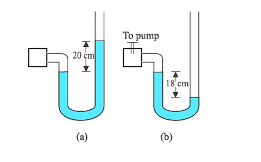Solution: For figure (a)
Atmospheric pressure, $P_{0}=76 \mathrm{~cm}$ of $\mathrm{Hg}$
Here gauge pressure is the difference between the levels of mercury in the two limbs. Hence, gauge pressure is $20 \mathrm{~cm}$ of $\mathrm{Hg}$.
Absolute pressure $=$ Atmospheric pressure $+$ Gauge pressure
$=76+20=96 \mathrm{~cm}$ of $\mathrm{Hg}$
For figure (b)
Difference between the levels of mercury in the two limbs $=-18 \mathrm{~cm}$
Hence, gauge pressure $=-18 \mathrm{~cm}$ of $\mathrm{Hg}$.
Absolute pressure $=$ Atmospheric pressure $+$ Gauge pressure
Absolute pressure $=76 \mathrm{~cm}-18 \mathrm{~cm}=58 \mathrm{~cm}$
$13.6 \mathrm{~cm}$ of water is poured into the right limb of figure (b).
The relative density of mercury $=13.6$
Hence, a column of $13.6 \mathrm{~cm}$ of water is equivalent to $1 \mathrm{~cm}$ of mercury.
(b) Let $h$ be the difference between the levels of mercury in the two limbs.
The pressure in the right limb is given as:
$P_{R}=$ Atmospheric pressure $+1 \mathrm{~cm}$ of $\mathrm{Hg}=76+1=77 \mathrm{~cm}$ of $\mathrm{Hg} \quad \ldots$ (i)
The level of mercury column will rise in the left limb.
Hence, pressure in the left limb, $P_{L}=58+h$... (ii)
Equating equations (i) and (ii), we get:
$77=58+h$
$\therefore h=19 \mathrm{~cm}$
Hence, the difference between the levels of mercury in the two limbs will be $19 \mathrm{~cm}$

$Question.23$ Two vessels have the same base area but different shapes. The first vessel takes twice the volume of water that the second vessel requires to fill up to a particular common height. Is the force exerted by the water on the base of the vessel the same in the two cases ? If so, why do the vessels filled with water to that same height give different readings on a weighing scale?

Solution: Yes
Equal force and equal pressure will act on the common base area of the two vessels having the same base area. Since the shapes of the two vessels are different, the force exerted on the sides of the vessels has non-zero vertical components. When these vertical components are added, the total force on one vessel comes out to be greater than that on the other vessel. Hence, when these vessels are filled with water to the same height, they give different readings on a weighing scale.

Question.24 During blood transfusion the needle is inserted in a vein where the gauge pressure is $2000 \mathrm{~Pa}$. At what height must the blood container be placed so that blood may just enter the vein? [Use the density of whole blood from Table].

Solution:
Given that gauge pressure, $P=2000 \mathrm{~Pa}$
From the table, the density of whole blood, $\rho =1.06 \times 10^{3} \mathrm{~kg} \mathrm{~m}^{-3}$
Acceleration due to gravity, $\mathrm{g} =9.8 \mathrm{~m} / \mathrm{s}^{2}$
Height of the blood container $=h$
The pressure of the blood container, $P=h \rho g$
$\therefore h=\frac{P}{\rho g}$
$\Rightarrow h=\frac{2000}{1.06 \times 10^{3} \times 9.8} \mathrm{~m}$
$\Rightarrow h=0.1925 \mathrm{~m}$
The blood will enter the vein if the blood container is kept at a height greater than $0.1925 \mathrm{~m}$, i.e., about $0.2 \mathrm{~m}$.

Question.25$In deriving Bernoulli's equation, we equated the work done on the fluid in the tube to its change in the potential and kinetic energy. (a) What is the largest average velocity of blood flow in an artery of diameter$2 \times 10^{-3} \mathrm{~m}$if the flow must remain laminar ? (b) Do the dissipative forces become more important as the fluid velocity increases? Discuss qualitatively. Solution: The diameter of the artery,$d=2 \times 10^{-3} \mathrm{~m}$The viscosity of blood,$\eta=2.084 \times 10^{-3} \mathrm{~Pa} \mathrm{~s}$The density of blood,$\rho=1.06 \times 103 \mathrm{~kg} / \mathrm{m}^{3}$Reynolds' number for laminar flow,$N_{R}=2000$The largest average velocity of blood is given as: Therefore, the largest average velocity of blood is$1.966 \mathrm{~m} / \mathrm{s}$. As the fluid velocity increases, the dissipative forces become more important. This is because of the rise of turbulence. Turbulent flow causes a dissipative loss in a fluid.$Question.26$(a) What is the largest average velocity of blood flow in an artery of radius$2 \times 10^{-3} \mathrm{~m}$if the flow must remain laminar? (b) What is the corresponding flow rate (Take viscosity of blood to be$\left.2.084 \times 10^{-3} \mathrm{~Pa} \mathrm{~s}\right)$Solution. The radius of the artery,$r=2 \times 10^{-3} \mathrm{~m}$The diameter of the artery,$d=2 \times 2 \times 10-3 \mathrm{~m}=4 \times 10^{-3} \mathrm{~m}$The viscosity of blood,$\eta=2.084 \times 10^{-3} \mathrm{~Pa} \mathrm{~s}$The density of blood,$\rho=1.06 \times 10^{3} \mathrm{~kg} / \mathrm{m}^{3}$Reynolds' number for laminar flow,$N_{R}=2000$The largest average velocity of blood is given by the relation:$V_{\operatorname{lrg}}=\frac{N_{R} \eta}{\rho d}\Rightarrow V_{\operatorname{lrg}}=\frac{2000 \times 2.084 \times 10^{-3}}{1.06 \times 10^{3} \times 4 \times 10^{-3}} \mathrm{~m} / \mathrm{s}\Rightarrow V_{\mathrm{lrg}}=0.983 \mathrm{~m} / \mathrm{s}\Rightarrow R=3.14 \times\left(2 \times 10^{-3}\right)^{2} \times 0.983 \mathrm{~m}^{3} \mathrm{~s}^{-1}\Rightarrow R=1.235 \times 10^{-5} \mathrm{~m}^{3} \mathrm{~s}^{-1}$Therefore, the corresponding flow rate is$1.235 \times 10^{-5} \mathrm{~m}^{3} \mathrm{~s}^{-1}Question.27$A plane is in level flight at a constant speed, and each of its two wings has an area of$25 \mathrm{~m}^{2}$. If the speed of the air is$180 \mathrm{~km} / \mathrm{h}$over the lower wing and$234 \mathrm{~km} / \mathrm{h}$over the upper wing surface, determine the plane's mass. (Take air density to be$1 \mathrm{~kg} \mathrm{~m}^{-3}$). Solution. The area of the wings of the plane,$A=2 \times 25=50 \mathrm{~m}^{2}$Speed of air over the lower wing,$V 1=180 \mathrm{~km} / \mathrm{h}=\frac{180 \times 1000}{3600}=50 \mathrm{~m} / \mathrm{s}$Speed of air over the upper wing,$V_{2}=234 \mathrm{~km} / \mathrm{h}=\frac{234 \times 1000}{3600}=65 \mathrm{~m} / \mathrm{s}$The density of air,$\rho=1 \mathrm{~kg} \mathrm{~m}^{-3}$The pressure of air over the lower wing$=P_{1}$The pressure of air over the upper wing$=P_{2}$The upward force on the plane can be obtained using Bernoulli's equation as:$P_{1}+\frac{1}{2} \rho V_{1}^{2}=P_{2}+\frac{1}{2} \rho V_{2}^{2}P_{1}-P_{2}=\frac{1}{2} \rho\left(V_{2}^{2}-V_{1}^{2}\right)$... (i) The upward force$(F)$on the plane can be calculated as:$\left(P_{1}-P_{2}\right) AF=\frac{1}{2} \rho\left(V_{2}^{2}-V_{1}^{2}\right) A$using equation (i)$F=\frac{1}{2} \times 1 \times\left((65)^{2}-(50)^{2}\right) \times 50 \mathrm{~N}F=43125 \mathrm{~N}$Using Newton's force equation, we can obtain the mass$(m)$of the plane as:$F=m g\therefore m=\frac{43125}{9.8}m=4400.51 \mathrm{~kg}$Hence, the mass of the plane is about$4400 \mathrm{~kg}$(approx). Question. 28 In Millikan's oil drop experiment, what is the terminal speed of an uncharged drop of radius$2.0 \times 10^{-5} \mathrm{~m}$and density$1.2 \times 10^{3} \mathrm{~kg} \mathrm{~m}^{-3}$. Take the viscosity of air at the temperature of the experiment to be$1.8 \times 10^{-5} \mathrm{~Pa} \mathrm{~s}$. How much is the viscous force on the drop at that speed ? Neglect buoyancy of the drop due to air. Solution: Terminal speed$=5.8 \mathrm{~cm} / \mathrm{s} ;$Viscous force$=3.9 \times 10^{-10} \mathrm{~N}$The radius of the given uncharged drop,$r=2.0 \times 10-5 \mathrm{~m}$The density of the uncharged drop,$\rho=1.2 \times 10^{3} \mathrm{~kg} \mathrm{~m}^{-3}$The viscosity of air,$\eta=1.8 \times 10^{-5} \mathrm{~Pa} \mathrm{~s}$Density of air$\left(\rho_{\mathrm{o}}\right)$can be taken as zero in order to neglect buoyancy of air. Acceleration due to gravity,$\mathrm{g}=9.8 \mathrm{~m} / \mathrm{s}^{2}$Terminal velocity$(v)$is given by the relation:$v=\frac{2 r^{2} \times\left(\rho-\rho_{\mathrm{o}}\right) \mathrm{g}}{9 \eta}\Rightarrow v=\frac{2 \times\left(2.0 \times 10^{-5}\right)^{2}\left(1.2 \times 10^{3}-0\right) \times 9.8}{9 \times 1.8 \times 10^{-5}} \mathrm{~m} \mathrm{~s}^{-1}\Rightarrow v=5.807 \times 10^{-2} \mathrm{~m} \mathrm{~s}^{-1}\Rightarrow v=5.8 \mathrm{~cm} \mathrm{~s}^{-1}$Hence, the terminal speed of the drop is$5.8 \mathrm{~cm} \mathrm{~s}^{-1}$. The viscous force on the drop is given by:$F=6 \pi \eta r v\therefore F=6 \times 3.14 \times 1.8 \times 10^{-5} \times 2.0 \times 10^{-5} \times 5.8 \times 10^{-2} \mathrm{~N}=3.9 \times 10^{-10} \mathrm{~N}$Hence, the viscous force on the drop is$3.9 \times 10^{-10} \mathrm{~N}$. Question.29 Mercury has an angle of contact equal to$140^{\circ}$with soda lime glass. A narrow tube of radius$1.00 \mathrm{~mm}$made of this glass is dipped in a trough containing mercury. By what amount does the mercury dip down in the tube relative to the liquid surface outside? The surface tension of mercury at the temperature of the experiment is$0.465 \mathrm{~N} \mathrm{~m}^{-1}$, the density of mercury$=13.6 \times 10^{3} \mathrm{~kg} \mathrm{~m}^{-3}$. Solution: The angle of contact between mercury and soda lime glass,$\theta=140^{\circ}$The radius of the narrow tube,$r=1 \mathrm{~mm}=1 \times 10^{-3} \mathrm{~m}$The surface tension of mercury at the given temperature,$S=0.465 \mathrm{~N} \mathrm{~m}^{-1}$The density of mercury,$\rho=13.6 \times 10^{3} \mathrm{~kg} / \mathrm{m}^{3}$Dip in the height of mercury$=h$Acceleration due to gravity,$\mathrm{g}=9.8 \mathrm{~m} / \mathrm{s}^{2}$Surface tension is related with the angle of contact and the dip in height as:$S=\frac{h \rho g r}{2 \cos \theta}\therefore h=\frac{2 S \cos \theta}{r \rho g}\Rightarrow h=\frac{2 \times 0.465 \times \cos 140}{1 \times 10^{-3} \times 13.6 \times 10^{3} \times 9.8} \mathrm{~m}\Rightarrow h=-0.00534 \mathrm{~m}\Rightarrow h=-5.34 \mathrm{~mm}$Here, the negative sign shows the decreasing level of mercury. Hence, the mercury level dips by$5.34 \mathrm{~mm}$. Qiuestion.30 Two narrow bores of diameters$3.0 \mathrm{~mm}$and$6.0 \mathrm{~mm}$are joined together to form a U-tube open at both ends. If the U-tube contains water, what is the difference in its levels in the two limbs of the tube ? The surface tension of water at the temperature of the experiment is$7.3 \times 10^{-2} \mathrm{~N} \mathrm{~m}^{-1}$. Take the angle of contact to be zero and density of water to be$1.0 \times 10^{3} \mathrm{~kg} \mathrm{~m}^{-3}\left(\mathrm{~g}=9.8 \mathrm{~m} \mathrm{~s}^{-2}\right)$. Solution. Given that the diameter of the first bore,$=3.0 \mathrm{~mm}=3 \times 10^{-3} \mathrm{~m}$Hence, the radius of the first bore,$r_{1}=\frac{d_{1}}{2}=1.5 \times 10^{-3} \mathrm{~m}$The diameter of the second bore,$d_{2}=6.0 \mathrm{~mm}$Hence, the radius of the second bore,$r_{2}=\frac{d_{2}}{2}=3 \times 10^{-3} \mathrm{~m}$The surface tension of water,$S=7.3 \times 10^{-2} \mathrm{~N} \mathrm{~m}^{-1}$The angle of contact between the bore surface and water,$\theta=0$The density of water,$\rho=1.0 \times 10^{3} \mathrm{~kg} / \mathrm{m}^{3}$Acceleration due to gravity,$\mathrm{g}=9.8 \mathrm{~m} / \mathrm{s}^{2}$Let$h_{1}$and$h_{2}$be the heights to which water rises in the first and second tubes respectively. These heights are given by the relations:$h_{1}=\frac{2 S \cos \theta}{r_{1} \rho g}\ldots$... (i)$h_{2}=\frac{2 S \cos \theta}{r_{2} \rho g}\ldots$(ii) Let$\mathrm{h}$is the difference between the levels of water in the two limbs of the tube.$h=\frac{2 S \cos \theta}{r_{1} \rho g}-\frac{2 S \cos \theta}{r_{2} \rho g}=\frac{2 S \cos \theta}{\rho g}\left[\frac{1}{r_{1}}-\frac{1}{r_{2}}\right]=\frac{2 \times 7.3 \times 10^{-2} \times 1}{1 \times 10^{3} \times 9.8}\left[\frac{1}{1.5 \times 10^{-3}}-\frac{1}{3 \times 10^{-3}}\right]=4.966 \times 10^{-3} \mathrm{~m}=4.97 \mathrm{~mm}$Hence, the difference between the levels of water in the two bores is$4.97 \mathrm{~mm}$. Question.31 (a) It is known that density$\mathrm{r}$of air decreases with height$\mathrm{y}$as$\rho=\rho_{0} \mathrm{e}^{-y / y_{0}}$where$\rho_{0}=1.25 \mathrm{~kg} \mathrm{~m}^{-3}$is the density at sea level, and$\mathrm{y}_{0}$is a constant. This density variation is called the law of atmospheres. Obtain this law assuming that the temperature of the atmosphere remains a constant (isothermal conditions). Also, assume that the value of$\mathrm{g}$remains constant. (b) A large He balloon of volume$1425 \mathrm{~m}^{3}$is used to lift a payload of$400 \mathrm{~kg}$. Assume that the balloon maintains constant radius as it rises. How high does it rise?$\left[\right.$Take$\mathrm{y}_{0}=8000 \mathrm{~m}$and$\left.\rho_{\mathrm{He}}=0.18 \mathrm{~kg} \mathrm{~m}^{-3}\right]$. Solution: Given that volume of the balloon,$V=1425 \mathrm{~m}^{3}$Mass of the payload,$m=400 \mathrm{~kg}$Acceleration due to gravity,$\mathrm{g}=9.8 \mathrm{~m} / \mathrm{s}^{2}y_{\mathrm{o}}=8000 \mathrm{~m}$(Given in the question)$\rho_{\mathrm{He}}=0.18 \mathrm{~kg} \mathrm{~m}^{-3}\rho_{\mathrm{o}}=1.25 \mathrm{~kg} \mathrm{~m}^{-3}$The density of the balloon$=\rho$Height to which the balloon rises$=y$Density$(\rho)$of air decreases with height$(y)$as:$\rho=\rho_{\mathrm{o}} e^{-y / y_{0}}\frac{\rho}{\rho_{\mathrm{o}}}=e^{-y / y_{0}}$... (i) This density variation is called the law of atmospherics. We can say from the equation (i) that the rate of decrease of density with height is directly proportional to$\rho$, i.e.,$-\frac{d \rho}{d y} \propto \rho\frac{d \rho}{d y}=-k \rhod \rho \backslash \rho=-k d y$Where$k$is the constant of proportionality Height changes from 0 to$\mathrm{y}$, while density changes from$\rho_{\mathrm{o}}$to$\rho$. Integrating the sides between these limits, we get:$\int_{\rho_{0}}^{\rho} \frac{d \rho}{\rho}=-\int_{0}^{y} k d y\left[\log _{e} \rho\right]_{\rho_{0}}^{\rho}=-k y\log _{e} \frac{\rho}{\rho_{\mathrm{o}}}=-k y\frac{\rho}{\rho_{\mathrm{o}}}=e^{-k y}\ldots(\mathrm{ii})$Comparing equations (i) and (ii), we get:$y_{\mathrm{o}}=\frac{1}{k}k=\frac{1}{y_{\mathrm{o}}} \ldots$(iii) From equations (i) and (iii), we get:$\rho=\rho_{\mathrm{o}}^{-y / y_{0}}$(b) Density$\rho=\frac{\text { Mass }}{\text { Volume }}\Rightarrow \rho=\frac{\text { Mass of the payload + Mass of helium }}{\text { Volume }}\Rightarrow \rho=\frac{\mathrm{m}+V \rho_{H e}}{V}\Rightarrow \rho=\frac{400+1425 \times 0.18}{1425} \mathrm{~kg} / \mathrm{m}^{3}\rho=0.46 \mathrm{~kg} / \mathrm{m}^{3}$From equations (ii) and (iii), we can obtain$y$as:$\rho=\rho_{\mathrm{o}} e^{-y / y_{0}}\log _{e} \frac{\rho}{\rho_{\mathrm{o}}}=-\frac{y}{y_{\mathrm{o}}}\therefore y=-8000 \times \log _{e} \frac{0.46}{1.25}\Rightarrow y=-8000 \times-1\Rightarrow y=8000 \mathrm{~m}=8 \mathrm{~km}$Hence, the balloon will rise to a height of$8 \mathrm{~km}\$.